# Overview

Quantitative real-time PCR is an important technique in medical and bio-medical applications. The pcr package provides a unified interface for quality assessing, analyzing and testing qPCR data for statistical significance. The aim of this document is to describe the different methods and modes used to relatively quantify gene expression of qPCR and their implementation in the pcr package.

# Getting started

The pcr is available on CRAN. To install it, use:

# install package CRAN
install.packages('pcr')

The development version of the package can be obtained through:

# install package from github
devtools::install_github('MahShaaban/pcr')
# load required libraries
library(pcr)

The following chunk of code locates a dataset of $$C_T$$ values of two genes from 12 different samples and performs a quick analysis to obtain the expression of a target gene c-myc normalized by a control GAPDH in the Kidney samples relative to the brain samples. pcr_analyze provides different methods, the default one that is used here is ‘delta_delta_ct’ applies the popular ($$\Delta\Delta C_T$$) method.

# default mode delta_delta_ct
## locate and read raw ct data
fl <- system.file('extdata', 'ct1.csv', package = 'pcr')

group_var <- rep(c('brain', 'kidney'), each = 6)

# calculate all values and errors in one step
## mode == 'separate_tube' default
res <- pcr_analyze(ct1,
group_var = group_var,
reference_gene = 'GAPDH',
reference_group = 'brain')

res

The output of pcr_analyze is explained in the documentation of the function ?pcr_analyze and the method it calls ?pcr_ddct as well as in a later scion of this document. Briefly, the input includes the $$C_T$$ value of c-myc normalized to the control GAPDH, The calibrated value of c-myc in the kidney relative to the brain samples and the final relative_expression of c-myc. In addition, an error term and a lower and upper intervals are provided.

The previous analysis makes a few assumptions that will be explained later in this document. One of which is a perfect amplification efficiency of the PCR reaction. To assess the validity of this assumption, pcr_assess provides a method called efficiency. The input data.frame is the $$C_T$$ values of c-myc and GAPDH at different input amounts/dilutions.

## locate and read data
fl <- system.file('extdata', 'ct3.csv', package = 'pcr')

## make a vector of RNA amounts
amount <- rep(c(1, .5, .2, .1, .05, .02, .01), each = 3)

## calculate amplification efficiency
res <- pcr_assess(ct3,
amount = amount,
reference_gene = 'GAPDH',
method = 'efficiency')
res

In the case of using the $$\Delta\Delta C_T$$, the assumption of the amplification efficiency is critical for the reliability of the model. In particular, the slope and the R^2 of the line between the log input amount and $$\Delta C_T$$ or the difference between the $$C_T$$ value of the target c-myc and GAPDH. Typically, The slope should be very small (less than 0.01). slope here is appropriate 0.0264562, A value of the amplification efficiency itself is given by $$10 ^ {-1/slope}$$, so the assumption holds true.

# Background

## Glossary

• Amplification efficiency: The ability of the reaction to amplify a certain amount of input RNA in a sample
• $$C_T$$: Cycle Threshold is the number of cycles required for the fluorescent signal to cross the threshold
• $$\Delta C_T$$: Difference between two $$C_T$$ values (e.g. $$C_{T, c-myc} - C_{T, GAPDH}$$)
• $$\Delta \Delta C_T$$: Difference between two $$\Delta C_T$$ values (e.g. $$\Delta C_{T, Treatment} - \Delta C_{T, Treatment}$$)
• Reference gene: A gene known not to change its expression between the groups of interest, so any change in its signal should be due to the amplification of the PCR. Used for normalization. (e.g. GAPDH or $$\beta$$-actin)
• Reference group: An experimental group used to express mRNA level in comparison to. Used for caliberation. (e.g. control or time point 0)
• Standard: A sample of known concentration

## Analysis methods

In contrast with the absolute quantification of the amount of mRNA in a sample, relative quantification uses a internal control (reference gene) and/or a control group (reference group) to quantify the mRNA of interest relative to these references. This relative quantification is sufficient to draw conclusions in most of the bio-medical applications involving qPCR. A few methods were developed to perform these relative quantification. These methods require different assumptions and models. The most common two of these methods are explained here.

### Comparative $$C_T$$ methods

The comparative $$C_T$$ methods assume that the cDNA templates of the gene/s of interest as well as the control/reference gene have similar amplification efficiency. And that this amplification efficiency is near perfect. Meaning, at a certain threshold during the linear portion of the PCR reaction, the amount of the gene of the interest and the control double each cycle. Another assumptions is that, the expression difference between two genes or two samples can be captured by subtracting one (gene or sample of interest) from another (reference). This final assumption requires also that these references doesn’t change with the treatment or the course in question.
The formal derivation of the double delta $$C_T$$ model is described here (Livak and Schmittgen 2001). Briefly,
The $$\Delta\Delta C_T$$ is given by:

$\Delta\Delta C_T = \Delta C_{T,q} - \Delta C_{T,cb}$

And the relative expression by:

$2^{-\Delta\Delta C_T}$

Where:

• $$\Delta C_{T,q}$$ is the difference in the $$C_T$$ (or their ) of a gene of interest and a reference gene in a group of interest
• $$\Delta C_{T,cb}$$ is the the difference in the $$C_T$$ (or their ) of a gene of interest and a reference gene in a reference group

And the error term is given by:

$s = \sqrt{s_1^2 + s_2^2}$

Where:

• $$s_1$$ is the of a gene of interest
• $$s_2$$ is the of a reference gene

### Standard curve methods

In comparison, this model doesn’t assume perfect amplification but rather actively use the amplification in calculating the relative expression. So when the amplification efficiency of all genes are 100% both methods should give similar results. The standard curve method is applied using two steps. First, serial dilutions of the mRNA from the samples of interest are used as input to the PCR reaction. The linear trend of the log input amount and the resulting $$C_T$$ values for each gene are used to calculate an intercept and a slope. Secondly, these intercepts and slopes are used to calculate the amounts of mRNA of the genes of interest and the control/reference in the samples of interest and the control sample/reference. These amounts are finally used to calculate the relative expression in a manner similar to the later method, just using division instead of subtraction.
The formal derivation of the model is described here (Yuan et al. 2006). Briefly,
The amount of RNA in a sample is given by:

$\log amount = \dfrac{C_T - b}{m}$

And the relative expression is given by:

$10^{\log amount}$

where:

• $$C_T$$ is the cycle threshold of a gene

• $$b$$ is the of $$C_T$$ ~ log10 input amount

• $$m$$ is the of $$C_T$$ ~ log10 input amount

And the error term is given by:

$s = (cv)(\bar X)$

Where:

$cv = \sqrt{cv_1^2 + cv_2^2}$

Where:

• $$s$$ is the

• $$\bar X$$ is the

• $$cv$$ is the or relative standard deviation

## Quality Assessment of qPCR

Fortunately, regardless of the method used in the analysis of qPCR data, The quality assessment are done in a similar way. It requires an experiment similar to that of calculating the standard curve. Serial dilutions of the genes of interest and controls are used as input to the reaction and different calculations are made.

• The amplification efficiency is approximated be the linear trend between the difference between the $$C_T$$ value of a gene of interest and a control/reference ($$\Delta C_T$$) and the log input amount. This piece of information is required when using the $$\Delta\Delta C_T$$ model. Typically, the slope of the curve should be very small and the $$R^2$$ value should be very close to one. A value of the amplification efficiency itself is given by $$10 ^ {-1/slope}$$ and should be close to 2. Other analysis methods are recommended when this is not the case.
• Similar curves are required for each gene using the $$C_T$$ value instead of the difference for applying the standard curve method. In this case, a separate slope and intercept are required for the calculation of the relative expression.

## Testing statistical significance

Using the later two methods and there assumptions, useful statistics such as p-values and confidence intervals can be calculated.

### Two-group tests

Assuming that the assumptions of the first methods are holding true, the simple t-test can be used to test the significance of the difference between two conditions ($$\Delta C_T$$). t-test assumes in addition, that the input $$C_T$$ values are normally distributed and the variance between conditions are comparable. Wilcoxon test can be used when sample size is small and those two last assumptions are hard to achieve.

### Linear regression

Two use the linear regression here. A null hypothesis is formulated as following,

$C_{T, target, treatment} - C_{T, control, treatment} = C_{T, target, control} - C_{T, control, control} \quad \textrm{or} \quad \Delta\Delta C_T$

This is exactly the ($$\Delta\Delta C_T$$) as explained earlier. So the $$\Delta\Delta C_T$$ is estimated and the null is rejected when $$\Delta\Delta C_T \ne 0$$.

# The pcr package

## Motivation

To illustrate the use of the pcr package in applying these methods on qPCR data, we use real qPCR real qPCR datasets from two published papers. In addition, we compare the results obtained by the pcr package to that of the original paper to ensure the reliability. First, Livak et al. (Livak and Schmittgen 2001) obtained total RNA from human tissues; brain and kidney. c-myc and GAPDH primers were then used for cDNA synthesis and used as input in the PCR reaction. 6 replicates for each tissue were run in separate sample. This dataset is referred to as ct1 through this document and is shown along with the difference calculations in Table 1 and 2. Another dataset was generated from separate assay. Only running the samples in the same tube this time with pairs of primates that has different reporting dyes. This is referred to as ct2 and is shown in Table 3 and 4. Finally, $$C_T$$ values from a qPCR experiment using different input amounts of c-myc and GAPDH was conducted. The dataset is referred to as ct3 and is shown in Table 5. Secondly, Yuan et al. (Yuan et al. 2006) extracted total RNA from Arabidopsis thaliana plant treated and control samples, 24 samples each. And performed a qPCR to using MT7 and ubiquitin primers. This dataset is referred to as ct4 and Table 6 shows the results of the different testing methods that were applied in the original paper.

Table 1: Relative quantification using comparative ($$\Delta\Delta C_T$$) method (separate tubes)
Tissue c-myc $$C_T$$ GAPDH $$C_T$$ $$\Delta C_T$$ (c-myc - GAPDH) $$\Delta\Delta C_T$$ ($$\Delta C_T - \Delta C_{T,Brain}$$) c-myc$$_N$$ (Rel. to Brain)
Brain 30.72 23.7
30.34 23.56
30.58 23.47
30.34 23.65
30.5 23.69
30.43 23.68
Average 30.49 $$\pm$$ 0.15 23.63 $$\pm$$ 0.09 6.86 $$\pm$$ 0.17 0.00 $$\pm$$ 0.17 1.0 (0.9–1.1)
Kidney 27.06 22.76
27.03 22.61
27.03 22.62
27.1 22.6
26.99 22.61
26.94 24.18
Average 27.03 $$\pm$$ 0.06 22.66 $$\pm$$ 0.08 4.37 $$\pm$$ 0.10 –2.50 $$\pm$$ 0.10 5.6 (5.3–6.0)
Table 2:Relative quantification using the standard curve method (separate tube)
Tissue c-myc (ng) GAPDH (ng) c-myc$$_N$$ (norm. to GAPDH) c-myc$$_N$$ (Rel. to Brain)
Brain 0.033 0.51
0.043 0.56
0.036 0.59
0.043 0.53
0.039 0.51
0.040 0.52
Average 0.039 $$\pm$$ 0.004 0.54 $$\pm$$ 0.034 0.07 $$\pm$$ 0.008 1.0 $$\pm$$ 0.12
Kidney 0.40 0.96
0.41 1.06
0.41 1.05
0.39 1.07
0.42 1.06
0.43 0.96
Average 0.41 $$\pm$$ 0.016 1.02 $$\pm$$ 0.052 0.40 $$\pm$$ 0.025 5.5 $$\pm$$ 0.35
Table 3: Relative quantification using comparative ($$\Delta\Delta C_T$$) method (same tube)
Tissue c-myc $$C_T$$ GAPDH $$C_T$$ $$\Delta C_T$$ (c-myc - GAPDH) $$\Delta\Delta C_T$$ ($$Ave.\Delta C_T - Ave. \Delta C_{T,Brain}$$) c-myc$$_N$$ (Rel. to Brain)
Brain 32.38 25.07 7.31
32.08 25.29 6.79
32.35 25.32 7.03
32.08 25.24 6.84
32.34 25.17 7.17
32.13 25.29 6.84
Average 6.99 $$\pm$$ 0.21 0.00 $$\pm$$ 0.21 1.0 (0.86–1.15)
Kidney 28.73 24.30 4.43
28.84 24.32 4.52
28.51 24.31 4.20
28.86 24.25 4.61
28.86 24.34 4.52
28.70 24.18 4.52
Average 4.47 $$\pm$$ 0.14 –2.53 $$\pm$$ 0.14 5.77 (5.23–6.37)
Table 4: Relative quantification using the standard curve method (same tube)
Tissue c-myc (ng) GAPDH (ng) c-myc$$_N$$ (norm. to GAPDH) c-myc$$_N$$ (Rel. to Brain)
Brain 0.031 0.618 0.05
0.038 0.532 0.07
0.032 0.521 0.06
0.038 0.550 0.07
0.032 0.577 0.06
0.037 0.532 0.07
Average 0.06 $$\pm$$ 0.008 1.0 $$\pm$$ 0.14
Kidney 0.365 0.049 0.35
0.338 1.035 0.33
0.423 1.042 0.41
0.334 1.086 0.31
0.334 1.021 0.33
0.372 1.139 0.33
Average 0.34 $$\pm$$ 0.035 5.4 $$\pm$$ 0.55
Table 5: Average $$C_T$$ value for c-myc and GAPDH at different input amounts
Input RNA (ng) c-myc (Average $$C_T$$) GAPDH (Average $$C_T$$) $$\Delta C_T$$ (c-myc - GAPDH)
1.0 25.59 $$\pm$$ 0.04 22.64 $$\pm$$ 0.03 2.95 $$\pm$$ 0.05
0.5 26.77 $$\pm$$ 0.09 23.73 $$\pm$$ 0.05 3.04 $$\pm$$ 0.10
0.2 28.14 $$\pm$$ 0.05 25.12 $$\pm$$ 0.10 3.02 $$\pm$$ 0.11
0.1 29.18 $$\pm$$ 0.13 26.16 $$\pm$$ 0.02 3.01 $$\pm$$ 0.13
0.05 30.14 $$\pm$$ 0.03 27.17 $$\pm$$ 0.06 2.97 $$\pm$$ 0.07
0.02 31.44 $$\pm$$ 0.16 28.62 $$\pm$$ 0.10 2.82 $$\pm$$ 0.19
0.02 32.42 $$\pm$$ 0.12 29.45 $$\pm$$ 0.08 2.97 $$\pm$$ 0.14
Table 6: Statistical significance using different testing methods
Test $$\Delta\Delta C_T$$ (estimate) p-value Confidence Interval
Multiple Regression -0.6848 0.0001 (-0.4435, -0.9262)
ANOVA -0.6848 0.0001 (-0.4435, -0.9262)
t-test -0.6848 0.0001 (-0.4147, -0.955)
Wilcoxon test -0.6354 0.0001 (-0.4227, -0.8805)

## Assess pcr_assess

pcr_assess is a wrapper for the implemented quality assessment methods; pcr_efficiency and pcr_standard. Both methods can be called directly using the method names or through pcr_assess by passing a string to the argument method; ‘efficiency’ or ‘standard_curve’ for calculating the amplification efficiency or the standard curve for each gene respectively.

### Amplification efficiency pcr_efficiency

To calculate the amplification efficiency in a qPCR experiment, the main input is a data.frame with columns contain the $$C_T$$ values for each gene and rows correspond to the different input amounts/dilutions (Table 5).

The following code apply the calculation on the data.frame, ct3. It has two columns c_myc and GAPDH and 3 rows for each of the input amounts coded in the variable amounts. A reference gene passed to the reference_gene argument, in this case, the column name GAPDH.

library(pcr)
library(ggplot2)
library(cowplot)
## locate and read data
fl <- system.file('extdata', 'ct3.csv', package = 'pcr')

## make a vector of RNA amounts
amount <- rep(c(1, .5, .2, .1, .05, .02, .01), each = 3)

## calculate amplification efficiency
res <- pcr_assess(ct3,
amount = amount,
reference_gene = 'GAPDH',
method = 'efficiency')
knitr::kable(res, caption = 'Table 7: amplification efficiency of c-myc')
Table 7: amplification efficiency of c-myc
gene intercept slope r_squared
c_myc 3.01448 0.0264562 0.0207027

The output of pcr_assess is a data.frame of 4 columns and n rows equals the input genes except for the reference. For each gene an intercept, slope and $$R^2$$ is calculated for a the difference between it and the reference ($$\Delta C_T$$) (Table 7).

When the argument plot is TRUE a graph is produced instead shows the average and standard deviation of of the $$\Delta C_T$$ at difference input amounts. In addition, a linear trend line is drawn (Fig 1).

gg <- pcr_assess(ct3,
amount = amount,
reference_gene = 'GAPDH',
method = 'efficiency',
plot = TRUE)
gg +
labs(x = 'log10 amount', y = 'Delta Ct') +
theme(strip.background = element_blank(),
strip.text = element_blank())
## Warning: Use of df$x is discouraged. Use x instead. ## Warning: Use of df$y is discouraged. Use y instead.
## Warning: Use of df$x is discouraged. Use x instead. ## Warning: Use of df$y is discouraged. Use y instead.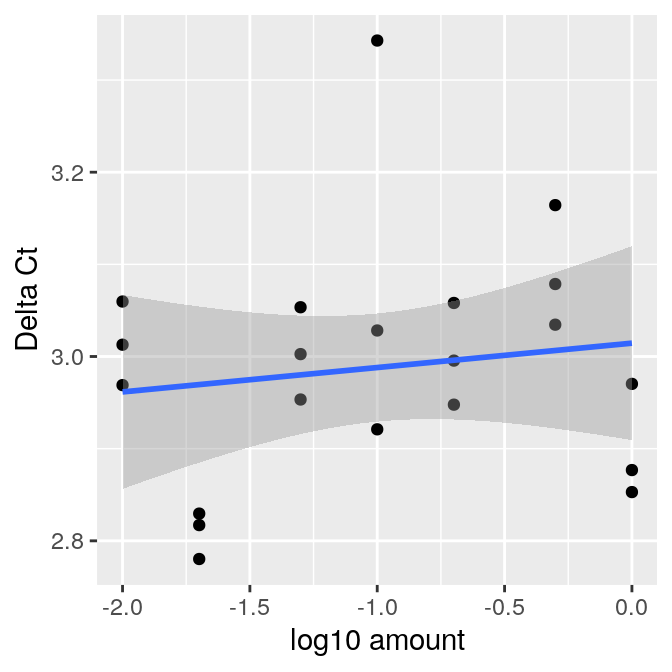Figure 1: Amplification efficiency of c-myc

The relevant summaries in calculating efficiency is the slope. Typically, slope should be very low (less than 0.1). Means the $$\Delta C_T$$ are not changing much as a consequence of changing the input concentration. A value of the amplification efficiency itself is given by $$10 ^ {-1/slope}$$ and should be close to 2.

### Standard curve pcr_standard

To calculate the standard curve for individual genes, pcr_assess takes a data.frame similar to that described above as input ct3, and the same amount variable. The following code calculates the curves for the two columns/genes by fitting a line between their $$C_T$$ values and the log input amounts.

## calculate standard curve
res <- pcr_assess(ct3,
amount = amount,
method = 'standard_curve')
knitr::kable(res, caption = 'Table 8: Standard curves of c-myc and GAPDH')
Table 8: Standard curves of c-myc and GAPDH
gene intercept slope r_squared
c_myc 25.69669 -3.388095 0.9965504
GAPDH 22.68221 -3.414551 0.9990278

The output put is similar to the previous call, except when ‘standard_curve’ is passed to method curves are calculated for individual genes, column gene (Table 8).

The information of the standard curves are required when using the standard curve methods, so we retain the relevant ones in the variables intercept and slope. Typically, the r_squared should be close to 1.

intercept <- res$intercept slope <- res$slope

When the argument plot is TRUE a graph is returned instead. A panel for each gene showing the raw $$C_T$$ values and the log input amounts (Fig. 2).

gg <- pcr_assess(ct3,
amount = amount,
method = 'standard_curve',
plot = TRUE)
gg +
labs(x = 'Log 10 amount', y = 'CT value')
## Warning: Use of df$x is discouraged. Use x instead. ## Warning: Use of df$y is discouraged. Use y instead.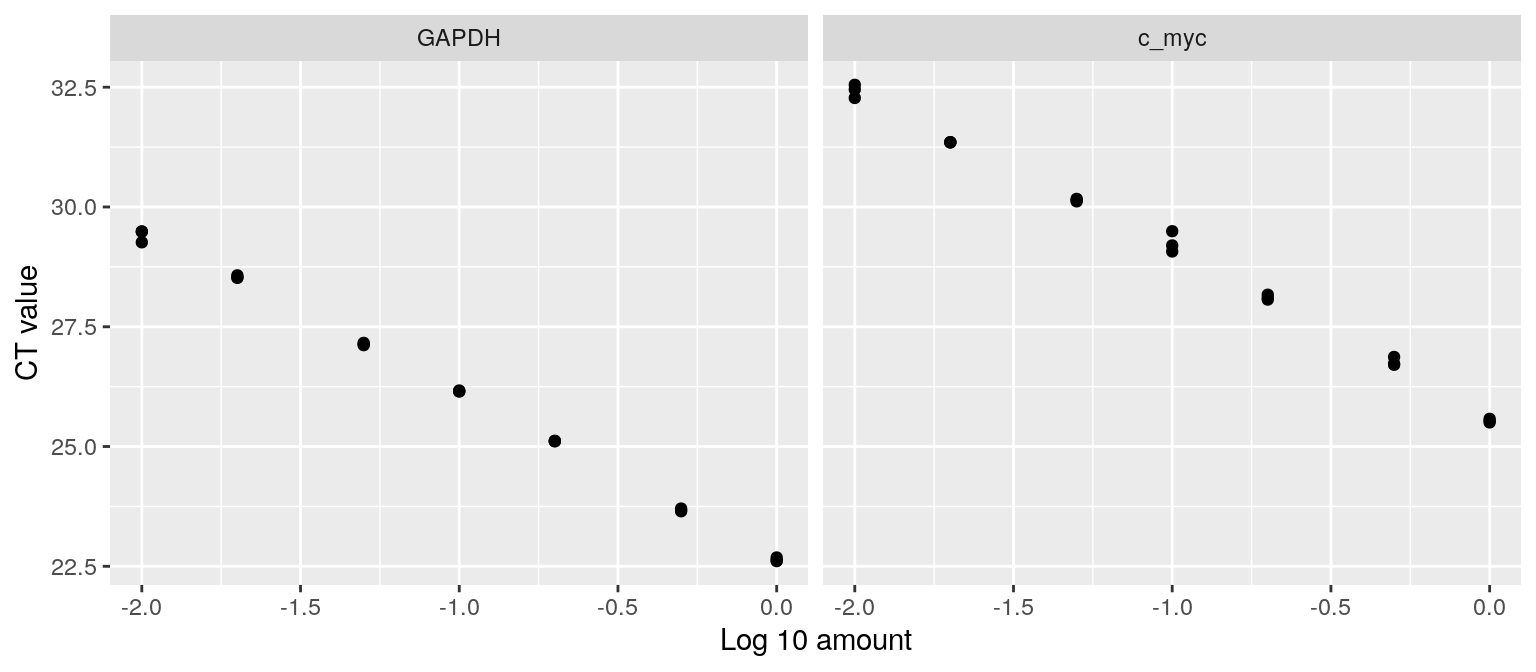Figure 2: Standard curve of c-myc and GAPDH

## Analyze pcr_analyze

Similarly, pcr_analyze is a wrapper and unified interface for difference analysis models; pcr_ddct, pcr_dct and pcr_curve. The models can be invoked by calling these functions directly or through the argument method to pcr_analyze. Possible input to the argument method are ‘delta_delta_ct’, ‘delta_ct’ and ‘relative_curve’ for calculating the double delta $$C_T$$, delta $$C_T$$ and the standard curve models respectively.

### Doube delta $$C_T$$ ($$\Delta\Delta C_T$$) pcr_ddct

To apply the double delta $$C_T$$ model, the default method of pcr_analyze, the main input is a data.frame with columns containing the genes and the rows the $$C_T$$ values from different samples. In addition, a group variable group_var corresponding to these rows/samples is required. Finally, a reference_gene and a reference_group are entered.

The following code chunk applies this method to a data.frame of 12 samples from 2 groups ct1 (Table 1).

# default mode delta_delta_ct
## locate and read raw ct data
fl <- system.file('extdata', 'ct1.csv', package = 'pcr')

group_var <- rep(c('brain', 'kidney'), each = 6)

# calculate all values and errors in one step
## mode == 'separate_tube' default
res1 <- pcr_analyze(ct1,
group_var = group_var,
reference_gene = 'GAPDH',
reference_group = 'brain')

knitr::kable(res1, caption = 'Table 9: Double delta $C_T$ method (separate tubes)')
Table 9: Double delta $$C_T$$ method (separate tubes)
group gene normalized calibrated relative_expression error lower upper
brain c_myc 6.860 0.000 1.000000 0.1739540 0.886410 1.128146
kidney c_myc 4.365 -2.495 5.637283 0.0954463 5.276399 6.022850

The output of pcr_analyze is 8 columns; contains the calculations of each gene in each group and the error terms (Table 9). This analysis uses the default mode, ‘separate_tube’ as the input dataset came for an experiment where the target c_myc and the control gene GAPDH were ran in separate tubes.

In contrast, the ct2 dataset, also shown in Table 3, came from an identical experiment except the samples were run in the same tube. So the following analysis invokes a different mode, ‘same_tube’.

# calculate all values and errors in one step
## mode == 'same_tube'
res2 <- pcr_analyze(ct2,
group_var = group_var,
reference_gene = 'GAPDH',
reference_group = 'brain',
mode = 'same_tube')

knitr::kable(res2, caption = 'Table 10: Double delta $C_T$ method (same tube)')
Table 10: Double delta $$C_T$$ method (same tube)
group gene normalized calibrated relative_expression error lower upper
brain c_myc 6.996667 0.00 1.000000 0.2103014 0.8643567 1.156930
kidney c_myc 4.466667 -2.53 5.775717 0.1425015 5.2324932 6.375336

The only difference here is that the average of the $$C_T$$ values for the target gene c_myc is calculated after normalizing by the reference gene GAPDH. The rest of the calculations are expected to be slightly different than the previous case (Table 10).

Figure 3 shows the results of these two analysis. Bars represent the average relative expression of c-myc in the kidney, normalized by GAPDH and calibrated by the brain. The error bars are the standard deviations.

gg1 <- pcr_analyze(ct1,
group_var = group_var,
reference_gene = 'GAPDH',
reference_group = 'brain',
plot = TRUE) +
labs(x = '', y = 'Relative mRNA expression') +
ggtitle(label = 'Separate tubes')
gg2 <- pcr_analyze(ct2,
group_var = group_var,
reference_gene = 'GAPDH',
reference_group = 'brain',
mode = 'same_tube',
plot = TRUE) +
labs(x = '', y = 'Relative mRNA expression') +
ggtitle(label = 'Same tubes')
plot_grid(gg1, gg2)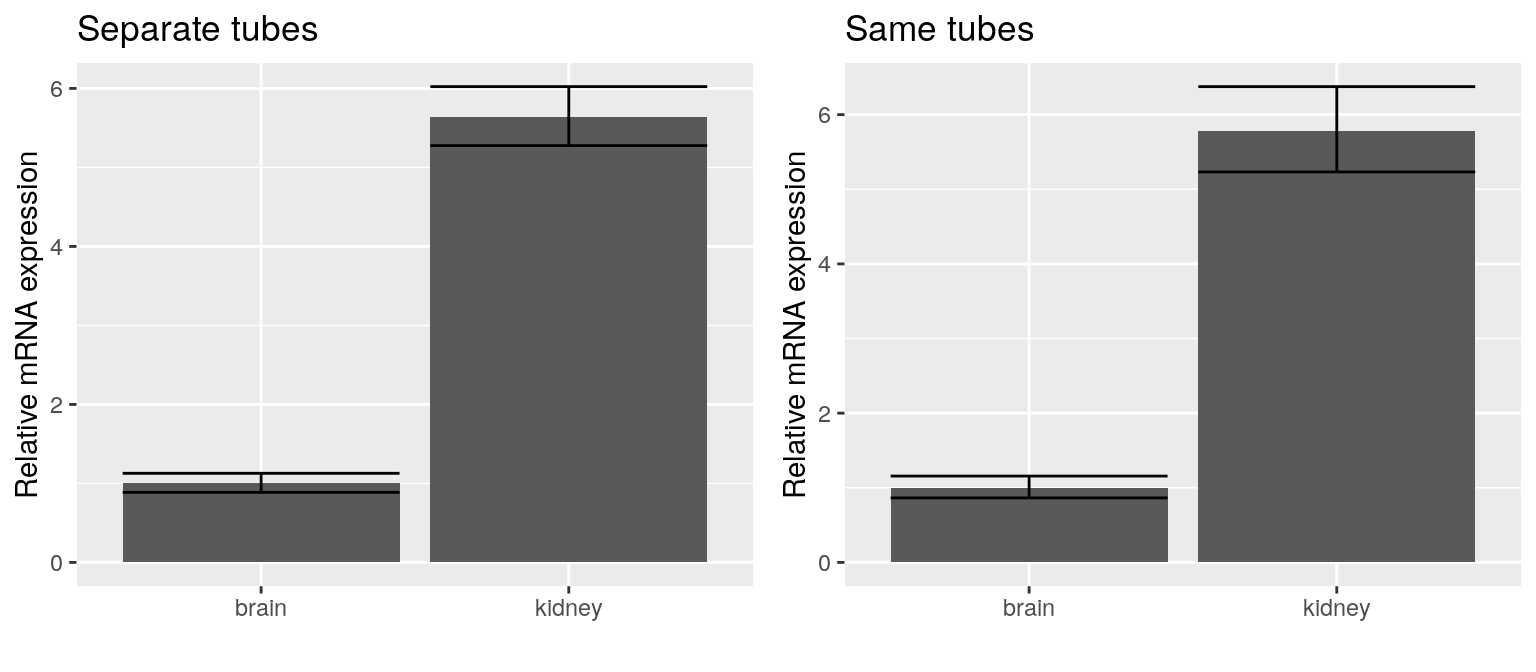Figure 3: Relative expression of c-myc using double delta $$C_T$$

### Delta $$C_T$$ ($$\Delta C_T$$) pcr_dct

This method is a variation of the double delta $$C_T$$ model. It can be used to calculate the fold change of in one sample relative to the others. For example, it can be used to compare and choosing a control/reference genes.

## example to check fold change of control gens
fl <- system.file('extdata', 'ct1.csv', package = 'pcr')

## make a data.frame of two identical columns
pcr_hk <- data.frame(
GAPDH1 = ct1$GAPDH, GAPDH2 = ct1$GAPDH
)

group_var <- rep(c('brain', 'kidney'), each = 6)

Here, we used the column GAPDH from the dataset ct1 to make a data.frame, pcr_hk of two identical columns GAPDH1 and GAPDH2 two show how such comparison can be done.

The input to pcr_analyze is identical to that of the previous call, only method is specified this time to ‘delta_ct’.

# delta_ct method
## calculate caliberation
res <- pcr_analyze(pcr_hk,
group_var = group_var,
reference_group = 'brain',
method = 'delta_ct')

knitr::kable(res, caption = 'Table 11: Delta $C_T$ method')
Table 11: Delta $$C_T$$ method
group gene calibrated fold_change error lower upper
brain GAPDH1 0.000 1.000000 0.0913783 0.9386256 1.065388
kidney GAPDH1 -0.965 1.952063 0.0777174 1.8496888 2.060104
brain GAPDH2 0.000 1.000000 0.0913783 0.9386256 1.065388
kidney GAPDH2 -0.965 1.952063 0.0777174 1.8496888 2.060104

Similarly, the output contains the calculated model and the error terms (Table 11). The difference here will be skipping the normalization step and calibrating the $$C_T$$ values of all genes to a reference_group.

Figure 4 shows the average relative fold change of the identical housekeeping genes and there error terms in two tissue samples.

pcr_analyze(pcr_hk,
group_var = group_var,
reference_group = 'brain',
method = 'delta_ct',
plot = TRUE) +
theme(legend.position = 'top',
legend.direction = 'horizontal') +
labs(x = '', y = 'Relative fold change')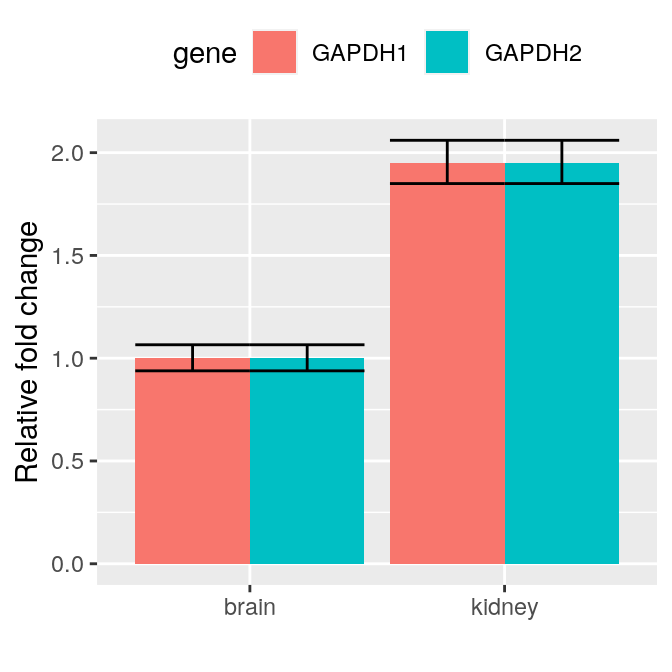Figure 4: GAPDH relative fold change using delta $$C_T$$

### Standard curve pcr_curve

The calculation of the standard curve method involves to steps as explained earlier. First, a standard curve is calculated for each gene to find the intercept and the slope. Then the relative expression is calculated.

To apply this method to the ct1 dataset (Table 2). We used the variables slope and intercept that were calculated earlier using the ct3 dataset (Table 8).

## calculate standard amounts and error
res1 <- pcr_analyze(ct1,
group_var = group_var,
reference_gene = 'GAPDH',
reference_group = 'brain',
intercept = intercept,
slope = slope,
method = 'relative_curve')

knitr::kable(res1, caption = 'Table 12: Standard curve method (separate tubes)')
Table 12: Standard curve method (separate tubes)
group gene normalized calibrated error lower upper
brain c_myc 0.0731034 1.000000 0.0085340 0.8832608 1.116739
kidney c_myc 0.3992171 5.460996 0.0255136 5.3970866 5.524905

The output of pcr_analyze is the same as explained before. The calculated averages and error term of target genes in each group relative to the reference gene and group (Table 12).

The argument mode can be can be used to change the way the $$C_T$$ values are averaged when samples from different genes were ran in the same tube (Table 4).

## calculate standard amounts and error
res2 <- pcr_analyze(ct2,
group_var = group_var,
reference_gene = 'GAPDH',
reference_group = 'brain',
intercept = intercept,
slope = slope,
method = 'relative_curve',
mode = 'same_tube')

knitr::kable(res2, caption = 'Table 13: Standard curve method (same tube)')
Table 13: Standard curve method (same tube)
group gene normalized calibrated error lower upper
brain c_myc 0.066439 1.000000 0.0091634 0.8620773 1.137923
kidney c_myc 0.371088 5.585394 0.0378101 5.4835041 5.687284

The output is similar to that described earlier (Table 13).

Figure 5 shows the output of the standard curve method. Relative expression values of c-myc in the kidney normalized by GAPDH and calibrated to the brain are shown as bars, Averages $$\pm$$ standard deviations.

gg1 <- pcr_analyze(ct1,
group_var = group_var,
reference_gene = 'GAPDH',
reference_group = 'brain',
intercept = intercept,
slope = slope,
method = 'relative_curve',
plot = TRUE) +
labs(x = '', y = 'Relative mRNA expression') +
ggtitle(label = 'Separate tubes')
gg2 <- pcr_analyze(ct2,
group_var = group_var,
reference_gene = 'GAPDH',
reference_group = 'brain',
intercept = intercept,
slope = slope,
method = 'relative_curve',
mode = 'same_tube',
plot = TRUE) +
labs(x = '', y = 'Relative mRNA expression') +
ggtitle(label = 'Same tubes')
plot_grid(gg1, gg2)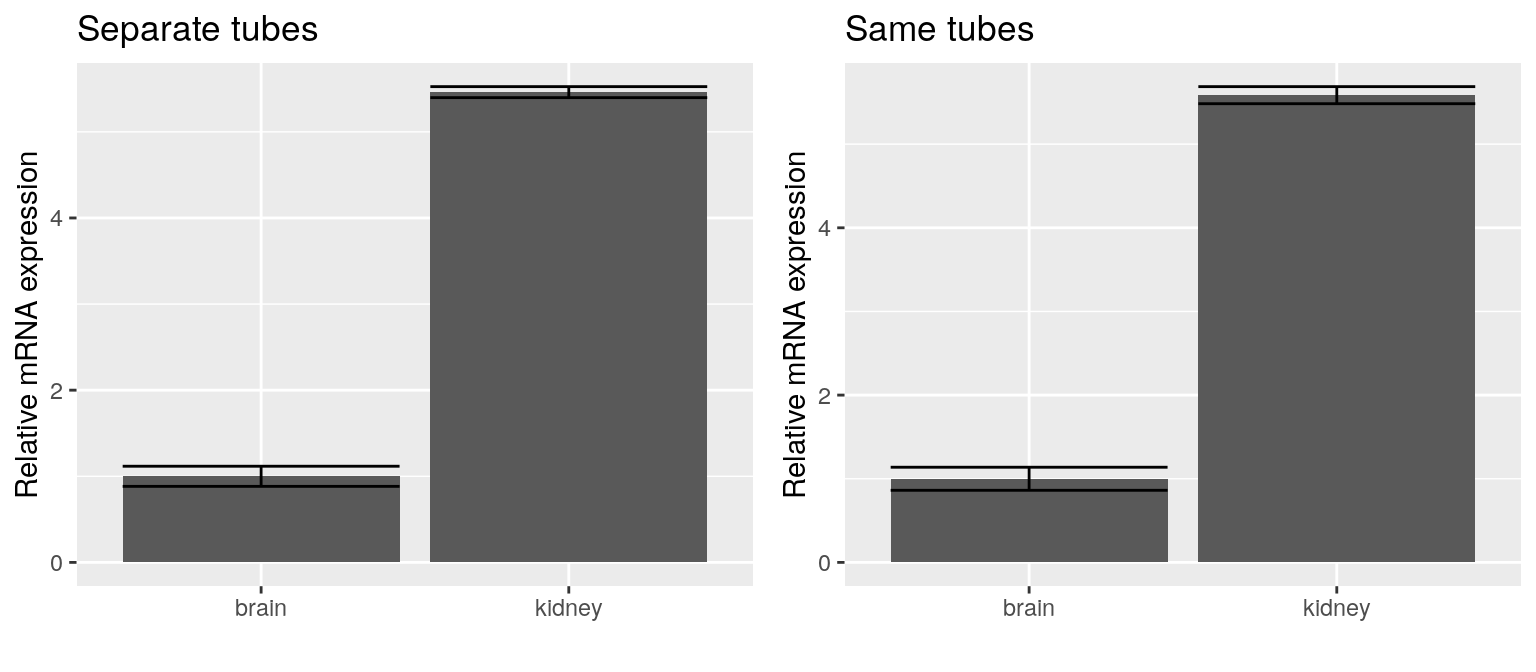Figure 5: Relative expression of c-myc using the standard curve

## Test pcr_test

Testing for statistical significance between conditions is important to ensure validity and replicability of the analysis. Different statistical methods require different assumptions. So the choice of which test to use depends on many factors. Among these factors are the number of the conditions/groups, the sample and replicate sizes and the type of desired comparison.

pcr_test provides a unified interface to different testing methods, which is similar to that used before for analysis and quality assessment.

Here, we used a dataset ct4 of 48 samples and two gene columns ref and target. The samples came from two groups as indicated in the variable group. arguments reference_gene and reference_control are used to construct the comparison the same way they were used to calculate the relative expression.

# locate and read data
fl <- system.file('extdata', 'ct4.csv', package = 'pcr')

# make group variable
group <- rep(c('control', 'treatment'), each = 12)
# analyze the testing data
res <- pcr_analyze(ct4,
group_var = group,
reference_gene = 'ref',
reference_group = 'control')
ggplot(res, aes(x = group, y = relative_expression)) +
geom_col() +
labs(x = '', y = 'Relative mRNA expression')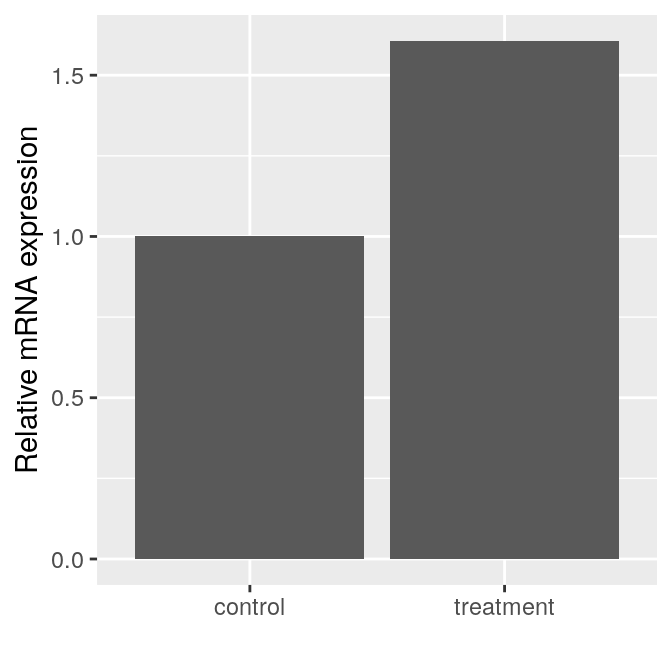Figure 6: Relative expression of target gene using delta delta $$C_T$$

We start by analyzing the dataset ct4 using the default method of pcr_analyze, ‘delta_delta_ct’ and show a bar graph of the results (Fig. 6).

Finally we used the pcr_test to perform different tests. The resulting output tables can be compared to the results from the original paper that provided the dataset (Table 6).

# test using t-test
tst1 <- pcr_test(ct4,
group_var = group,
reference_gene = 'ref',
reference_group = 'control',
test = 't.test')

knitr::kable(tst1, caption = 'Table 14: t-test summary')
Table 14: t-test summary
gene estimate p_value lower upper
target -0.684825 3.43e-05 -0.955952 -0.413698

When the argument test is set to ‘t.test’ a simple two-group t-test is carried out and an estimate for the difference between groups for the change in the target relative to a control gene is provided. In addition, a p_vale, a lower and upper 95% confidence intervals are provided as well (Table 14).

# test using wilcox.test
tst2 <- pcr_test(ct4,
group_var = group,
reference_gene = 'ref',
reference_group = 'control',
test = 'wilcox.test')

knitr::kable(tst2, caption = 'Table 15: Wilcoxon test summary')
Table 15: Wilcoxon test summary
gene estimate p_value lower upper
target -0.6354 3e-06 -0.8805 -0.4227

When the argument test is set to ‘wilcox.test’, Wilcoxon test method is used instead and similar output is provided (Table 15).

The linear regression can be applied to more than two groups and more advanced comparisons.

# testing using lm
tst3 <- pcr_test(ct4,
group_var = group,
reference_gene = 'ref',
reference_group = 'control',
test = 'lm')

knitr::kable(tst3, caption = 'Table 16: Linear regression summary')
Table 16: Linear regression summary
gene term estimate p_value lower upper
target group_vartreatment -0.684825 2.83e-05 -0.9549519 -0.4146981

The output of the test, ‘lm’ contains an extra column term to show the different comparison terms used to calculate the results (Table 16).

# Comparison with existing pacakges

Pabinger et al. surveyed the tools used to analyze qPCR data across different platforms (Pabinger et al. 2014). They included 9 R packages which provide very useful analysis and visualization methods. Some of these packages focuses one certain models and some are designed to handle high-throughput qPCR data. Most of these packages are hosted in CRAN and a few on the Bioconductor so they adhere to Bioconductor methods and data containers. In comparison, pcr provides a unified interface for different quality assessment, analysis and testing models. The input and the output are tidy data.frame, and the package source code follows the tidy-verse practices. This package is targets the small scale qPCR experimental data and there R user practitioner. The interface and documentation choices were made with such users in mind and require no deep knowledge in specific data structures or complex statistical models.

# Miscellaneous

In this section, we discuss briefly some common issues that arises when using real-time qPCR data and some simplified solutions using the pcr package. Mainly, we use linear models to quantify the effect of variables external to the reaction conditions on the resulting data and analysis. Either to identify such effects or to inform further experiments that are more likely to yield better results once the sources of the problems were removed. The examples are applied to the ct4 dataset alone with some artificial variable.

## Testing advanced desgins and hypotheses

When considering testing, multiple variable in an experiment or their interactions the argument model_matrix should be used in pcr_test. The model_matrix should be constructed first to reflect the hypothesis at hand. For example, when having multiple dose of a treatment. A vector of numerical values is constructed first to indicate the dose that were used with each sample. Along with the main grouping variable group, they should be combined in a data.frame and the function model.matrix is used. The first argument to this function is the formula of the comparison.

# testing advanced designs using a model matrix
# make a model matrix
group <- relevel(factor(group), ref = 'control')
dose <- rep(c(100, 80, 60, 40), each = 3, times = 2)
mm <- model.matrix(~group:dose, data = data.frame(group, dose))

# test using t-test
res <- pcr_test(ct4,
reference_gene = 'ref',
model_matrix = mm,
test = 'lm')

knitr::kable(res, caption = "Table 17: Testing advanced hypotheses")
gene term estimate p_value lower upper
target model_matrixgroupcontrol:dose 0.0048448 0.1752664 -0.0023371 0.0120266
target model_matrixgrouptreatment:dose -0.0035766 0.3121407 -0.0107585 0.0036053

In this case, the estimate effect of the interaction term between dose and group is very small and the p_value is very large (Table 17).

## Varying RNA quality among samples

The quality of the RNA is very critical and should be measured and pass the minimum threshold. Including the scaled qualities , quality, can be added to an interaction term in a linear model, to rule out its effect on the analysis if suspected to have any.

# using linear models to check the effect of RNA quality
# make a model matrix
group <- relevel(factor(group), ref = 'control')
set.seed(1234)
quality <- scale(rnorm(n = 24, mean = 1.9, sd = .1))
mm <- model.matrix(~group + group:quality, data = data.frame(group, quality))

# testing using lm
res <- pcr_test(ct4,
reference_gene = 'ref',
model_matrix = mm,
test = 'lm')

knitr::kable(res, caption = "Table 18: Check the effect of varying RNA quality")
Table 18: Check the effect of varying RNA quality
gene term estimate p_value lower upper
target model_matrixgrouptreatment -0.6561369 0.0000301 -0.9113989 -0.4008748
target model_matrixgroupcontrol:quality -0.2093469 0.0301799 -0.3964965 -0.0221973
target model_matrixgrouptreatment:quality 0.0865875 0.3321284 -0.0951329 0.2683078

The randomly generated quality seems to influence the results as indicated by the big estimate for the term ‘model_matrixgroupcontrol:quality’ and its p_value (Table 18).

## Combining data from multiple runs

The questions of whether it’s permissible to combine data from multiple runs depends on many factors. However, in practice it might be the only available option. In that case, one way to ensure the reliability of the data, is to use consider carefully which samples to run each time. Randomization or blocking can be used to avoid that batch effect or at least leave a chance to detect it if exists.

# using linear model to check the effects of mixing separate runs
# make a model matrix
group <- relevel(factor(group), ref = 'control')
run <- factor(rep(c(1:3), 8))
mm <- model.matrix(~group + group:run, data = data.frame(group, run))

# test using lm
res <- pcr_test(ct4,
reference_gene = 'ref',
model_matrix = mm,
test = 'lm')

knitr::kable(res, caption = "Table 19: Combining data from multiple qPCR runs")
Table 19: Combining data from multiple qPCR runs
gene term estimate p_value lower upper
target model_matrixgrouptreatment -0.681075 0.0126696 -1.1979712 -0.1641788
target model_matrixgroupcontrol:run2 -0.057975 0.8163730 -0.5748712 0.4589212
target model_matrixgrouptreatment:run2 -0.103625 0.6786077 -0.6205212 0.4132712
target model_matrixgroupcontrol:run3 -0.129725 0.6044471 -0.6466212 0.3871712
target model_matrixgrouptreatment:run3 -0.095325 0.7029678 -0.6122212 0.4215712

Here, we constructed a variable run to simulate a situation in which a dataset was generated in three separate runs. By including run in a model_matrix, its effect can be estimated. In this case, the estimate are very small and the p_value are very large, so it can be ignored (Table 19).

# Citation

citation("pcr")

# Contribution

## Bug reporting

• To report a bug please use the issue page on github

## Code contributions

• Fork this repo to your github account
• Clone the repo to your machine and make changes